# periodogram

Periodogram power spectral density estimate

## Syntax

pxx = periodogram(x)
pxx = periodogram(x,window)
pxx = periodogram(x,window,nfft)
[pxx,w] = periodogram(___)
[pxx,f] = periodogram(___,fs)
[pxx,w] = periodogram(x,window,w)
[pxx,f] = periodogram(x,window,f,fs)
[___] = periodogram(x,window,___,freqrange)
[___,pxxc] = periodogram(___,'ConfidenceLevel',probability)
[rpxx,f] = periodogram(___,'reassigned')
[rpxx,f,pxx,fc] = periodogram(___,'reassigned')
[___] = periodogram(___,spectrumtype)
periodogram(___)

## Description

example

pxx = periodogram(x) returns the periodogram power spectral density (PSD) estimate, pxx, of the input signal, x, found using a rectangular window. When x is a vector, it is treated as a single channel. When x is a matrix, the PSD is computed independently for each column and stored in the corresponding column of pxx. If x is real-valued, pxx is a one-sided PSD estimate. If x is complex-valued, pxx is a two-sided PSD estimate. The number of points, nfft, in the discrete Fourier transform (DFT) is the maximum of 256 or the next power of two greater than the signal length.

example

pxx = periodogram(x,window) returns the modified periodogram PSD estimate using the window, window. window is a vector the same length as x.

example

pxx = periodogram(x,window,nfft) uses nfft points in the discrete Fourier transform (DFT). If nfft is greater than the signal length, x is zero-padded to length nfft. If nfft is less than the signal length, the signal is wrapped modulo nfft and summed using datawrap. For example, the input signal [1 2 3 4 5 6 7 8] with nfft equal to 4 results in the periodogram of sum([1 5; 2 6; 3 7; 4 8],2).
[pxx,w] = periodogram(___) returns the normalized frequency vector, w. If pxx is a one-sided periodogram, w spans the interval [0,π] if nfft is even and [0,π) if nfft is odd. If pxx is a two-sided periodogram, w spans the interval [0,2π).

example

[pxx,f] = periodogram(___,fs) returns a frequency vector, f, in cycles per unit time. The sample rate, fs, is the number of samples per unit time. If the unit of time is seconds, then f is in cycles/second (Hz). For real-valued signals, f spans the interval [0,fs/2] when nfft is even and [0,fs/2) when nfft is odd. For complex-valued signals, f spans the interval [0,fs). fs must be the fourth input to periodogram. To input a sample rate and still use the default values of the preceding optional arguments, specify these arguments as empty, [].

example

[pxx,w] = periodogram(x,window,w) returns the two-sided periodogram estimates at the normalized frequencies specified in the vector, w. w must contain at least two elements, because otherwise the function interprets it as nfft.

example

[pxx,f] = periodogram(x,window,f,fs) returns the two-sided periodogram estimates at the frequencies specified in the vector. The vector f must contain at least two elements, because otherwise the function interprets it as nfft. The frequencies in f are in cycles per unit time. The sample rate, fs, is the number of samples per unit time. If the unit of time is seconds, then f is in cycles/second (Hz).

example

[___] = periodogram(x,window,___,freqrange) returns the periodogram over the frequency range specified by freqrange. Valid options for freqrange are: 'onesided', 'twosided', or 'centered'.

example

[___,pxxc] = periodogram(___,'ConfidenceLevel',probability) returns the probability × 100% confidence intervals for the PSD estimate in pxxc. 
[rpxx,f] = periodogram(___,'reassigned') reassigns each PSD estimate to the frequency closest to its center of energy. rpxx contains the sum of the estimates reassigned to each element of f.

example

[rpxx,f,pxx,fc] = periodogram(___,'reassigned') also returns the nonreassigned PSD estimates, pxx, and the center-of-energy frequencies, fc. If you use the 'reassigned' flag, then you cannot specify a probability confidence interval.

example

[___] = periodogram(___,spectrumtype) returns the PSD estimate if spectrumtype is specified as 'psd' and returns the power spectrum if spectrumtype is specified as 'power'.

example

periodogram(___) with no output arguments plots the periodogram PSD estimate in dB per unit frequency in the current figure window.

## Examples

collapse all

Obtain the periodogram of an input signal consisting of a discrete-time sinusoid with an angular frequency of $\pi /4$ rad/sample with additive $N\left(0,1\right)$ white noise.

Create a sine wave with an angular frequency of $\pi /4$ rad/sample with additive $N\left(0,1\right)$ white noise. The signal is 320 samples in length. Obtain the periodogram using the default rectangular window and DFT length. The DFT length is the next power of two greater than the signal length, or 512 points. Because the signal is real-valued and has even length, the periodogram is one-sided and there are 512/2+1 points.

n = 0:319; x = cos(pi/4*n)+randn(size(n)); [pxx,w] = periodogram(x); plot(w,10*log10(pxx))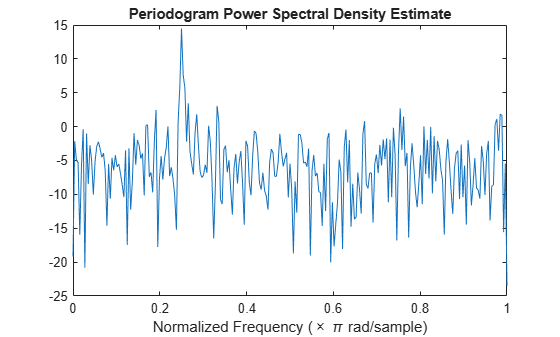Repeat the plot using periodogram with no outputs.

periodogram(x)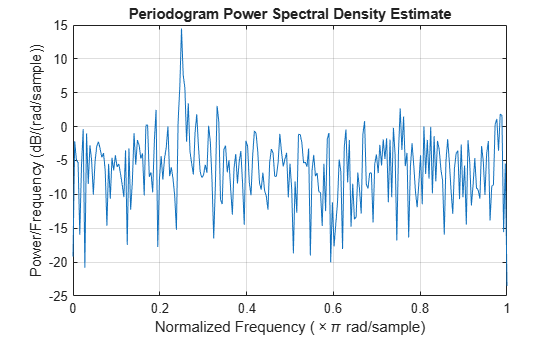Obtain the modified periodogram of an input signal consisting of a discrete-time sinusoid with an angular frequency of $\pi /4$ radians/sample with additive $N\left(0,1\right)$ white noise.

Create a sine wave with an angular frequency of $\pi /4$ radians/sample with additive $N\left(0,1\right)$ white noise. The signal is 320 samples in length. Obtain the modified periodogram using a Hamming window and default DFT length. The DFT length is the next power of two greater than the signal length, or 512 points. Because the signal is real-valued and has even length, the periodogram is one-sided and there are 512/2+1 points.

n = 0:319; x = cos(pi/4*n)+randn(size(n)); periodogram(x,hamming(length(x)))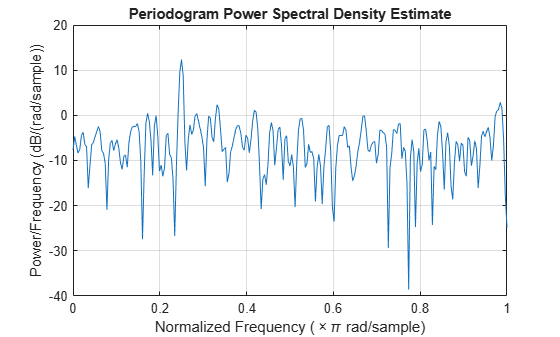Obtain the periodogram of an input signal consisting of a discrete-time sinusoid with an angular frequency of $\pi /4$ radians/sample with additive $N\left(0,1\right)$ white noise. Use a DFT length equal to the signal length.

Create a sine wave with an angular frequency of $\pi /4$ radians/sample with additive $N\left(0,1\right)$ white noise. The signal is 320 samples in length. Obtain the periodogram using the default rectangular window and DFT length equal to the signal length. Because the signal is real-valued, the one-sided periodogram is returned by default with a length equal to 320/2+1.

n = 0:319; x = cos(pi/4*n)+randn(size(n)); nfft = length(x); periodogram(x,[],nfft)Obtain the periodogram of the Wolf (relative sunspot) number data sampled yearly between 1700 and 1987.

Load the relative sunspot number data. Obtain the periodogram using the default rectangular window and number of DFT points (512 in this example). The sample rate for these data is 1 sample/year. Plot the periodogram.

load sunspot.dat relNums=sunspot(:,2); [pxx,f] = periodogram(relNums,[],[],1); plot(f,10*log10(pxx)) xlabel('Cycles/Year') ylabel('dB / (Cycles/Year)') title('Periodogram of Relative Sunspot Number Data')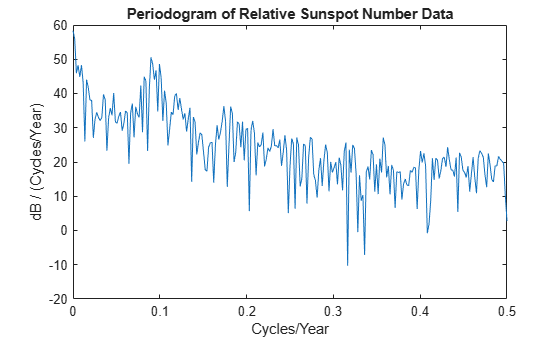You see in the preceding figure that there is a peak in the periodogram at approximately 0.1 cycles/year, which indicates a period of approximately 10 years.

Obtain the periodogram of an input signal consisting of two discrete-time sinusoids with an angular frequencies of $\pi /4$ and $\pi /2$ rad/sample in additive $N\left(0,1\right)$ white noise. Obtain the two-sided periodogram estimates at $\pi /4$ and $\pi /2$ rad/sample. Compare the result to the one-sided periodogram.

n = 0:319; x = cos(pi/4*n)+0.5*sin(pi/2*n)+randn(size(n)); [pxx,w] = periodogram(x,[],[pi/4 pi/2]); pxx
pxx = 1×2 14.0589 2.8872 
[pxx1,w1] = periodogram(x); plot(w1/pi,pxx1,w/pi,2*pxx,'o') legend('pxx1','2 * pxx') xlabel('\omega / \pi')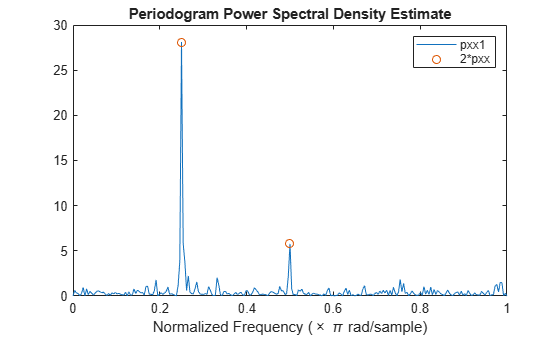The periodogram values obtained are 1/2 the values in the one-sided periodogram. When you evaluate the periodogram at a specific set of frequencies, the output is a two-sided estimate.

Create a signal consisting of two sine waves with frequencies of 100 and 200 Hz in N(0,1) white additive noise. The sampling frequency is 1 kHz. Obtain the two-sided periodogram at 100 and 200 Hz.

fs = 1000; t = 0:0.001:1-0.001; x = cos(2*pi*100*t)+sin(2*pi*200*t)+randn(size(t)); freq = [100 200]; pxx = periodogram(x,[],freq,fs)
pxx = 1×2 0.2647 0.2313 

The following example illustrates the use of confidence bounds with the periodogram. While not a necessary condition for statistical significance, frequencies in the periodogram where the lower confidence bound exceeds the upper confidence bound for surrounding PSD estimates clearly indicate significant oscillations in the time series.

Create a signal consisting of the superposition of 100 Hz and 150 Hz sine waves in additive white N(0,1) noise. The amplitude of the two sine waves is 1. The sampling frequency is 1 kHz.

fs = 1000; t = 0:1/fs:1-1/fs; x = cos(2*pi*100*t) + sin(2*pi*150*t) + randn(size(t));

Obtain the periodogram PSD estimate with 95%-confidence bounds. Plot the periodogram along with the confidence interval and zoom in on the frequency region of interest near 100 and 150 Hz.

[pxx,f,pxxc] = periodogram(x,rectwin(length(x)),length(x),fs,... 'ConfidenceLevel',0.95); plot(f,10*log10(pxx)) hold on plot(f,10*log10(pxxc),'-.') xlim([85 175]) xlabel('Hz') ylabel('dB/Hz') title('Periodogram with 95%-Confidence Bounds')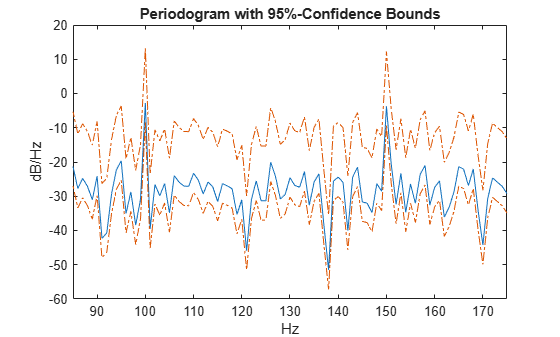The lower confidence bound in the immediate vicinity of 100 and 150 Hz is significantly above the upper confidence bound outside the vicinity of 100 and 150 Hz.

Obtain the periodogram of a 100 Hz sine wave in additive $N\left(0,1\right)$ noise. The data are sampled at 1 kHz. Use the 'centered' option to obtain the DC-centered periodogram and plot the result.

fs = 1000; t = 0:0.001:1-0.001; x = cos(2*pi*100*t)+randn(size(t)); periodogram(x,[],length(x),fs,'centered')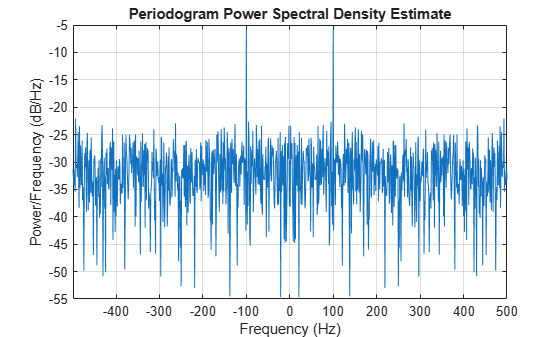Generate a signal that consists of a 200 Hz sinusoid embedded in white Gaussian noise. The signal is sampled at 1 kHz for 1 second. The noise has a variance of 0.01². Reset the random number generator for reproducible results.

rng('default') Fs = 1000; t = 0:1/Fs:1-1/Fs; N = length(t); x = sin(2*pi*t*200)+0.01*randn(size(t));

Use the FFT to compute the power spectrum of the signal, normalized by the signal length. The sinusoid is in-bin, so all the power is concentrated in a single frequency sample. Plot the one-sided spectrum. Zoom in to the vicinity of the peak.

q = fft(x,N); ff = 0:Fs/N:Fs-Fs/N; ffts = q*q'/N^2
ffts = 0.4997 
ff = ff(1:floor(N/2)+1); q = q(1:floor(N/2)+1); stem(ff,abs(q)/N,'*') axis([190 210 0 0.55])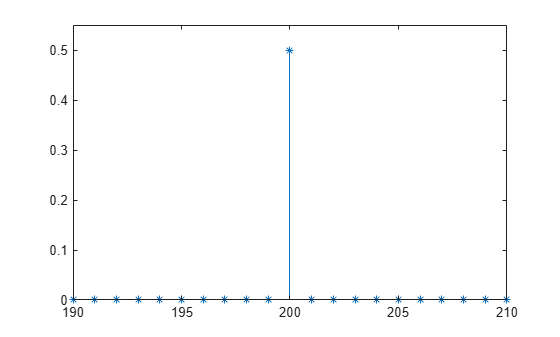Use periodogram to compute the power spectrum of the signal. Specify a Hann window and an FFT length of 1024. Find the percentage difference between the estimated power at 200 Hz and the actual value.

wind = hann(N); [pun,fr] = periodogram(x,wind,1024,Fs,'power'); hold on stem(fr,pun)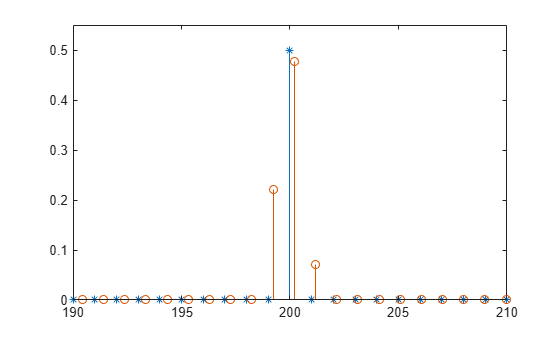periodogErr = abs(max(pun)-ffts)/ffts*100
periodogErr = 4.7349 

Recompute the power spectrum, but this time use reassignment. Plot the new estimate and compare its maximum with the FFT value.

[pre,ft,pxx,fx] = periodogram(x,wind,1024,Fs,'power','reassigned'); stem(fx,pre) hold off legend('Original','Periodogram','Reassigned')reassignErr = abs(max(pre)-ffts)/ffts*100
reassignErr = 0.0779 

Estimate the power of sinusoid at a specific frequency using the 'power' option.

Create a 100 Hz sinusoid one second in duration sampled at 1 kHz. The amplitude of the sine wave is 1.8, which equates to a power of 1.8²/2 = 1.62. Estimate the power using the 'power' option.

fs = 1000; t = 0:1/fs:1-1/fs; x = 1.8*cos(2*pi*100*t); [pxx,f] = periodogram(x,hamming(length(x)),length(x),fs,'power'); [pwrest,idx] = max(pxx); fprintf('The maximum power occurs at %3.1f Hz\n',f(idx))
The maximum power occurs at 100.0 Hz 
fprintf('The power estimate is %2.2f\n',pwrest)
The power estimate is 1.62 

Generate 1024 samples of a multichannel signal consisting of three sinusoids in additive $N\left(0,1\right)$ white Gaussian noise. The sinusoids' frequencies are $\pi /2$, $\pi /3$, and $\pi /4$ rad/sample. Estimate the PSD of the signal using the periodogram and plot it.

N = 1024; n = 0:N-1; w = pi./[2;3;4]; x = cos(w*n)' + randn(length(n),3); periodogram(x)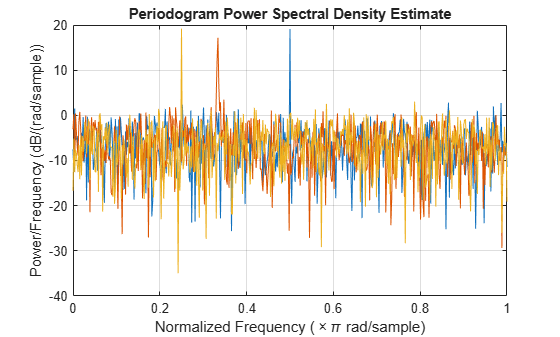Create a function periodogram_data.m that returns the modified periodogram power spectral density (PSD) estimate of an input signal using a window. The function specifies a number of discrete Fourier transform points equal to the length of the input signal.

type periodogram_data
function [pxx,f] = periodogram_data(inputData,window) %#codegen nfft = length(inputData); [pxx,f] = periodogram(inputData,window,nfft); end 

Use codegen (MATLAB Coder) to generate a MEX function.

• The %#codegen directive in the function indicates that the MATLAB® code is intended for code generation.

• The -args option specifies example arguments that define the size, class, and complexity of the inputs to the MEX-file. For this example, specify inputData as a 1024-by-1 double precision random vector and window as a Hamming window of length 1024. In subsequent calls to the MEX function, use 1024-sample input signals and windows.

• If you want the MEX function to have a different name, use the -o option.

• If you want to view a code generation report, add the -report option at the end of the codegen command.

codegen periodogram_data -args {randn(1024,1),hamming(1024)}
Code generation successful. 

Compute the PSD estimate of a 1024-sample noisy sinusoid using the periodogram function and the MEX function you generated. Specify a sinusoid normalized frequency of $2\pi /5$ rad/sample and a Hann window. Plot the two estimates to verify they coincide.

N = 1024; x = 2*cos(2*pi/5*(0:N-1)') + randn(N,1); periodogram(x,hann(N)) [pxMex,fMex] = periodogram_data(x,hann(N)); hold on plot(fMex/pi,pow2db(pxMex),':','Color',[0 0.4 0]) hold off grid on legend('periodogram','MEX function')## Input Arguments

collapse all

Input signal, specified as a row or column vector, or as a matrix. If x is a matrix, then its columns are treated as independent channels.

Example: cos(pi/4*(0:159))+randn(1,160) is a single-channel row-vector signal.

Example: cos(pi./[4;2]*(0:159))'+randn(160,2) is a two-channel signal.

Data Types: single | double
Complex Number Support: Yes

Window, specified as a row or column vector with the same length as the input signal. If you specify window as empty, then periodogram uses a rectangular window. If you specify the 'reassigned' flag and an empty window, then the function uses a Kaiser window with β = 38.

Data Types: single | double

Number of DFT points, specified as a positive integer. For a real-valued input signal, x, the PSD estimate, pxx has length (nfft/2 + 1) if nfft is even, and (nfft + 1)/2 if nfft is odd. For a complex-valued input signal,x, the PSD estimate always has length nfft. If nfft is specified as empty, the default nfft is used.

Data Types: single | double

Sample rate, specified as a positive scalar. The sample rate is the number of samples per unit time. If the unit of time is seconds, then the sample rate has units of Hz.

Normalized frequencies, specified as a row or column vector with at least two elements. Normalized frequencies are in rad/sample.

Example: w = [pi/4 pi/2]

Data Types: double

Frequencies, specified as a row or column vector with at least two elements. The frequencies are in cycles per unit time. The unit time is specified by the sample rate, fs. If fs has units of samples/second, then f has units of Hz.

Example: fs = 1000; f = [100 200]

Data Types: double

Frequency range for the PSD estimate, specified as a one of 'onesided', 'twosided', or 'centered'. The default is 'onesided' for real-valued signals and 'twosided' for complex-valued signals. The frequency ranges corresponding to each option are

• 'onesided' — returns the one-sided PSD estimate of a real-valued input signal, x. If nfft is even, pxx has length nfft/2 + 1 and is computed over the interval [0,π] rad/sample. If nfft is odd, the length of pxx is (nfft + 1)/2 and the interval is [0,π) rad/sample. When fs is optionally specified, the corresponding intervals are [0,fs/2] cycles/unit time and [0,fs/2) cycles/unit time for even and odd length nfft respectively.

The function multiplies the power by 2 at all frequencies except 0 and the Nyquist frequency to conserve the total power.

• 'twosided' — returns the two-sided PSD estimate for either the real-valued or complex-valued input, x. In this case, pxx has length nfft and is computed over the interval [0,2π) rad/sample. When fs is optionally specified, the interval is [0,fs) cycles/unit time.

• 'centered' — returns the centered two-sided PSD estimate for either the real-valued or complex-valued input, x. In this case, pxx has length nfft and is computed over the interval (–π,π] rad/sample for even length nfft and (–π,π) rad/sample for odd length nfft. When fs is optionally specified, the corresponding intervals are (–fs/2, fs/2] cycles/unit time and (–fs/2, fs/2) cycles/unit time for even and odd length nfft respectively.

Power spectrum scaling, specified as 'psd' or 'power'. To return the power spectral density, omit spectrumtype or specify 'psd'. To obtain an estimate of the power at each frequency, use 'power' instead. Specifying 'power' scales each estimate of the PSD by the equivalent noise bandwidth of the window, except when the 'reassigned' flag is used.

Coverage probability for the true PSD, specified as a scalar in the range (0,1). The output, pxxc, contains the lower and upper bounds of the probability × 100% interval estimate for the true PSD.

## Output Arguments

collapse all

PSD estimate, returned as a real-valued, nonnegative column vector or matrix. Each column of pxx is the PSD estimate of the corresponding column of x. The units of the PSD estimate are in squared magnitude units of the time series data per unit frequency. For example, if the input data is in volts, the PSD estimate is in units of squared volts per unit frequency. For a time series in volts, if you assume a resistance of 1 Ω and specify the sample rate in hertz, the PSD estimate is in watts per hertz.

Data Types: single | double

Cyclical frequencies, returned as a real-valued column vector. For a one-sided PSD estimate, f spans the interval [0,fs/2] when nfft is even and [0,fs/2) when nfft is odd. For a two-sided PSD estimate, f spans the interval [0,fs). For a DC-centered PSD estimate, f spans the interval (–fs/2, fs/2] cycles/unit time for even length nfft and (–fs/2, fs/2) cycles/unit time for odd length nfft.

Data Types: double | single

Normalized frequencies, returned as a real-valued column vector. If pxx is a one-sided PSD estimate, w spans the interval [0,π] if nfft is even and [0,π) if nfft is odd. If pxx is a two-sided PSD estimate, w spans the interval [0,2π). For a DC-centered PSD estimate, w spans the interval (–π,π] for even nfft and (–π,π) for odd nfft.

Data Types: double

Confidence bounds, returned as a matrix with real-valued elements. The row size of the matrix is equal to the length of the PSD estimate, pxx. pxxc has twice as many columns as pxx. Odd-numbered columns contain the lower bounds of the confidence intervals, and even-numbered columns contain the upper bounds. Thus, pxxc(m,2*n-1) is the lower confidence bound and pxxc(m,2*n) is the upper confidence bound corresponding to the estimate pxx(m,n). The coverage probability of the confidence intervals is determined by the value of the probability input.

Data Types: single | double

Reassigned PSD estimate, returned as a real-valued, nonnegative column vector or matrix. Each column of rpxx is the reassigned PSD estimate of the corresponding column of x.

Center-of-energy frequencies, specified as a vector or matrix.

collapse all

### Periodogram

The periodogram is a nonparametric estimate of the power spectral density (PSD) of a wide-sense stationary random process. The periodogram is the Fourier transform of the biased estimate of the autocorrelation sequence. For a signal xn sampled at fs samples per unit time, the periodogram is defined as

$\stackrel{^}{P}\left(f\right)=\frac{\Delta t}{N}\text{\hspace{0.17em}}\text{\hspace{0.17em}}{|\sum _{n=0}^{N-1}{x}_{n}{e}^{-j2\pi f\Delta t\text{\hspace{0.17em}}n}|}^{2},\text{\hspace{0.17em}}\text{\hspace{0.17em}}\text{\hspace{0.17em}}\text{ }-1/2\Delta t

where Δt is the sampling interval. For a one-sided periodogram, the values at all frequencies except 0 and the Nyquist, 1/2Δt, are multiplied by 2 so that the total power is conserved.

If the frequencies are in radians/sample, the periodogram is defined as

$\stackrel{^}{P}\left(\omega \right)=\frac{1}{2\pi N}\text{\hspace{0.17em}}\text{\hspace{0.17em}}{|\sum _{n=0}^{N-1}{x}_{n}{e}^{-j\omega n}|}^{2},\text{\hspace{0.17em}}\text{\hspace{0.17em}}\text{\hspace{0.17em}}\text{ }-\pi <\omega \le \pi .$

The frequency range in the preceding equations has variations depending on the value of the freqrange argument. See the description of freqrange in Input Arguments.

The integral of the true PSD, P(f), over one period, 1/Δt for cyclical frequency and 2π for normalized frequency, is equal to the variance of the wide-sense stationary random process:

${\sigma }^{2}={\int }_{-1/2\Delta t}^{1/2\Delta t}P\left(f\right)\text{\hspace{0.17em}}df.$

For normalized frequencies, replace the limits of integration appropriately.

### Modified Periodogram

The modified periodogram multiplies the input time series by a window function. A suitable window function is nonnegative and decays to zero at the beginning and end points. Multiplying the time series by the window function tapers the data gradually on and off and helps to alleviate the leakage in the periodogram. See Bias and Variability in the Periodogram for an example.

If hn is a window function, the modified periodogram is defined by

$\stackrel{^}{P}\left(f\right)=\frac{\Delta t}{N}\text{\hspace{0.17em}}\text{\hspace{0.17em}}{|\sum _{n=0}^{N-1}{h}_{n}{x}_{n}{e}^{-j2\pi f\Delta t\text{\hspace{0.17em}}n}|}^{2},\text{\hspace{0.17em}}\text{\hspace{0.17em}}\text{\hspace{0.17em}}\text{ }-1/2\Delta t

where Δt is the sampling interval.

If the frequencies are in radians/sample, the modified periodogram is defined as

$\stackrel{^}{P}\left(\omega \right)=\frac{1}{2\pi N}\text{\hspace{0.17em}}\text{\hspace{0.17em}}{|\sum _{n=0}^{N-1}{h}_{n}{x}_{n}{e}^{-j\omega n}|}^{2},\text{\hspace{0.17em}}\text{\hspace{0.17em}}\text{\hspace{0.17em}}\text{ }-\pi <\omega \le \pi .$

The frequency range in the preceding equations has variations depending on the value of the freqrange argument. See the description of freqrange in Input Arguments.

### Reassigned Periodogram

The reassignment technique sharpens the localization of spectral estimates and produces periodograms that are easier to read and interpret. This technique reassigns each PSD estimate to the center of energy of its bin, away from the bin’s geometric center. It provides exact localization for chirps and impulses.

 Auger, François, and Patrick Flandrin. "Improving the Readability of Time-Frequency and Time-Scale Representations by the Reassignment Method." IEEE® Transactions on Signal Processing. Vol. 43, May 1995, pp. 1068–1089.

 Fulop, Sean A., and Kelly Fitz. "Algorithms for computing the time-corrected instantaneous frequency (reassigned) spectrogram, with applications." Journal of the Acoustical Society of America. Vol. 119, January 2006, pp. 360–371.